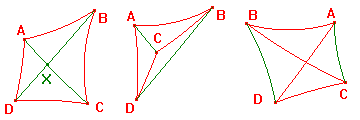Definition If A, B, C and D are points of D0 with no three on an h-line, then the h-quadrilateral ABCD consists of the h-segments AB, BC, CD and DA. The CabriJava window on the right allows you to experiment. By dragging the vertices, you should be able to see that there are essentially three types of h-quadrilateral. In the figure, we have added the h-segments AC and BD. These are the diagonals of ABCD. Observe that these may or may not meet. The three types are illustrated below.The first type is called convex. It is characterized by the fact that the h-segments AC and BD (the diagonals) intersect. Note that hyperbolic transformations preserve incidence, so that convex h-quadrilaterals map to convex h-quadrilaterals. For a convex h-quadrilateral, either diagonal divides the figure into two h-triangles. Since the sum of angles of an h-triangle is less than π, we have The h-quadrilateral Theorem The sum of internal angles of a convex h-quadrilateral is less than 2π. return to main hyperbolic menu